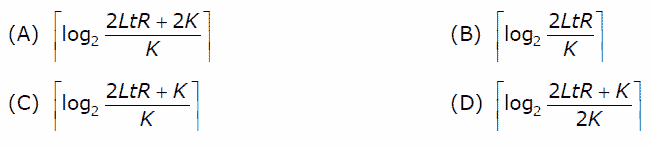Computer Networks | Set 10

• Difficulty Level : Hard
• Last Updated : 27 Mar, 2017

Following questions have been asked in GATE CS 2007 exam.

1) The address of a class B host is to be split into subnets with a 6-bit subnet number. What is the maximum number of subnets and the maximum number of hosts in each subnet?
(A) 62 subnets and 262142 hosts.
(B) 64 subnets and 262142 hosts.
(C) 62 subnets and 1022 hosts.
(D) 64 subnets and 1024 hosts.

Maximum number of subnets = 2^6-2 =62.
Note that 2 is subtracted from 2^6. The RFC 950 specification reserves the subnet values consisting of all zeros (see above) and all ones (broadcast), reducing the number of available subnets by two.

Maximum number of hosts is 2^10-2 = 1022.
2 is subtracted for Number of hosts is also. The address with all bits as 1 is reserved as broadcast address and address with all host id bits as 0 is used as network address of subnet.
In general, the number of addresses usable for addressing specific hosts in each network is always 2^N – 2 where N is the number of bits for host id.
See this for details

2) The message 11001001 is to be transmitted using the CRC polynomial x^3 + 1 to protect it from errors. The message that should be transmitted is:
(A) 11001001000
(B) 11001001011
(C) 11001010
(D) 110010010011

The polynomial x^3+1 corresponds to divisor is 1001.

11001001 000  <--- input right padded by 3 bits
1001          <--- divisor
01011001 000  <---- XOR of the above 2
1001         <--- divisor
00010001 000
1001
00000011 000
10 01
00000001 010
1 001
00000000 011 <------- remainder (3 bits)

See this for division process.
After dividing the given message 11001001 by 1001, we get the remainder as 011 which is the CRC. The transmitted data is, message + CRC which is 11001001 011.

3) The distance between two stations M and N is L kilometers. All frames are K bits long. The propagation delay per kilometer is t seconds. Let R bits/second be the channel capacity. Assuming that processing delay is negligible, the minimum number of bits for the sequence number field in a frame for maximum utilization, when the sliding window protocol is used, is:Distance between stations = L KM
Propogation delay per KM = t seconds
Total propagation delay = Lt seconds

Frame size = k bits
Channel capacity = R bits/second
Transmission Time = k/R

Let n be the window size.

UtiliZation = n/(1+2a) where a = Propagation time / transmission time
= n/[1 + 2LtR/k]
= nk/(2LtR+k)
For maximum utilization: nk = 2LtR + k
Therefore, n = (2LtR+k)/k
Number of bits needed for n frames is Logn.

See this for details.

4) Match the following:

(P) SMTP     (1) Application layer
(Q) BGP      (2) Transport layer
(R) TCP      (3) Data link layer
(S) PPP      (4) Network layer
(5) Physical layer

(A) P – 2 Q – 1 R – 3 S – 5
(B) P – 1 Q – 4 R – 2 S – 3
(C) P – 1 Q – 4 R – 2 S – 5
(D) P – 2 Q – 4 R – 1 S – 3

SMTP is an application layer protocol used for e-mail transmission.
TCP is a core transport layer protocol.
BGP is a network layer protocol backing the core routing decisions on the Internet
PPP is a data link layer protocol commonly used in establishing a direct connection between two networking nodes.

Please see GATE Corner for all previous year paper/solutions/explanations, syllabus, important dates, notes, etc.